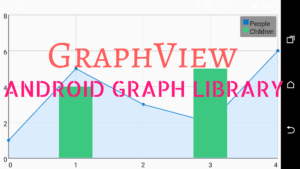How to add Data to GraphView from Arrays? [SOLVED] – Android StudioThanks to the amazing library by jjoe, you can plot graphs easily using GraphView.

The documentation provided is really helpful.

However, some users may still need more examples.

In this post I am gonna show you how to add Data to the GraphView from Arrays and Array Lists, or in other words how to plot data stored inside arrays or arrayLists.

Let’s say you have two arrayLists: x_axis and y_axis which contain the x and y axis data-points respectively:

```public static ArrayList<String> x_axis=new ArrayList<String>();
public static ArrayList<String> y_axis=new ArrayList<String>();
```

Or, similarly you could have two arrays x[n] and y[n] which contain the x and y data-points respectively:

```double[] x= new double[n];
double[] y= new double[n];
```

where n is the no. of data-pairs.

Now the next part deals with how to add the data from the arraylists to the GraphView. For this to work you would need to have the following dependency in your gradle file:

```dependencies{
compile 'com.jjoe64:graphview:4.2.1'
}
```

and also the graphView in your layout:

``` <com.jjoe64.graphview.GraphView
android:layout_width="match_parent"
android:layout_height="200dip"
android:id="@+id/graph"/>
```

To add data from arraylists that we created before, add the following code inside your OnCreate Method:

```GraphView graph;
PointsGraphSeries<DataPoint> series;       //an Object of the PointsGraphSeries for plotting scatter graphs
graph = (GraphView) findViewById(R.id.graph);
series= new PointsGraphSeries<>(data());   //initializing/defining series to get the data from the method 'data()'
series.setShape(PointsGraphSeries.Shape.POINT);
series.setSize(5);
```

The following is the definition of the method ‘data()’ which returns the DataPoint[] for the graph. You need to have this function definition in your activity.

```public DataPoint[] data(){
int n=x_axis.size();     //to find out the no. of data-points
DataPoint[] values = new DataPoint[n];     //creating an object of type DataPoint[] of size 'n'
for(int i=0;i<n;i++){
DataPoint v = new DataPoint(Double.parseDouble(x_axis.get(i)),Double.parseDouble(y_axis.get(i)));
values[i] = v;
}
return values;
}
```

The above code will plot the points from x_axis and y_axis arrayLists.

To plot the points from the arrays, change the above code as shown:

```public DataPoint[] data(){
DataPoint[] values = new DataPoint[n];     //creating an object of type DataPoint[] of size 'n'
for(int i=0;i<n;i++){
DataPoint v = new DataPoint(x[i],y[i]);
values[i] = v;
}
return values;
}
```